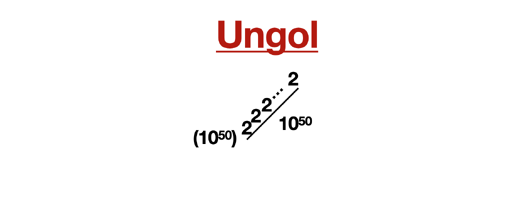11,055 PagesUngol in zeroes

The ungol is equal to $$r(10^{50},1)$$ in Rampant Array Notation or gogol squared a gogol times. The number was coined by Googology Wiki user Nirvana Supermind. It is larger than Skewe's Number but smaller than Googolduplex.

## Etymology

The name is derived from googol and the prefix un- meaning 1.

## Approximations

Notation Approximation
Scientific notation $$10^{10^{3.01\cdot{10^{49}}}}$$
Up-arrow notation $$(10 \uparrow 50) \uparrow {2 \uparrow (10 \uparrow 50)}$$ (exact value)
Rampant Array Notation r(10^50,1) (exact value)
BEAF {{10,50},{2,{10,50}}} (exact value)
Hyperfactorial Array Notation 40!!!
Fast-growing hierarchy $$f_2^{3}(158)$$

## See also

Basic notation:
Community content is available under CC-BY-SA unless otherwise noted.